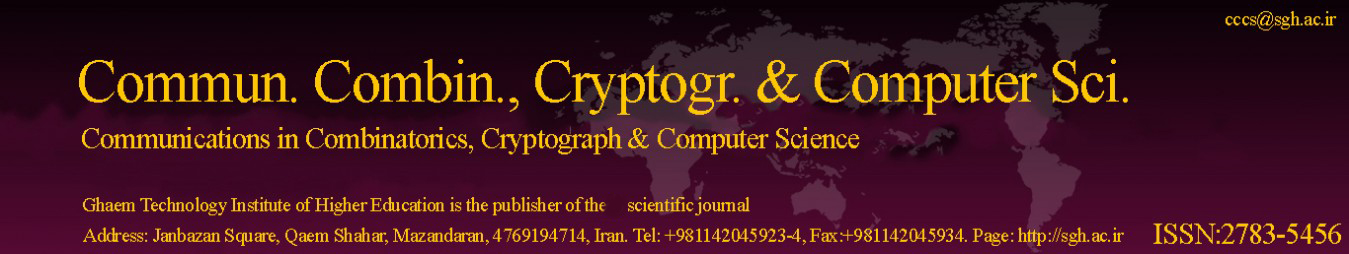Copy the following BibTeX for the article entitled A Public-Key Cryptographic Model Based on Hybrid Key Exchange Technique using Affine-Hill Cipher.

BibTeX

@article{Article_37,

title = {A Public-Key Cryptographic Model Based on Hybrid Key Exchange Technique using Affine-Hill Cipher},

journal = {Communications in Combinatorics, Cryptography & Computer Science},

volume = {2022},

issue = {2},

issn = { 2783-5456 },

year = {2022},

url = {http://cccs.sgh.ac.ir/Articles/2022/issue 2/2-1-SomeConjecturesonAverageofFibonacciandLucasSequences.pdf},

author = {Kshitish Kumar Mohanta and Deena Sunil Sharanappa},

keywords = {Public key cryptography, Elliptic Curve Diffie-Hellman(ECDH) Algorithm, ElGamal Algorithm, Generalized Fibonacci matrix, Affine-Hill Cipher.},

abstract = {In this paper, a hybrid method for creating key matrices using the multi-key exchange algorithm was suggested. It combines the ElGamal and Elliptic Curve Diffe Hellman (ECDH) algorithms. The key matrix for encryption and decryption uses the generalized Fibonacci matrix modulo p and the inverse of the generalized Fibonacci matrix modulo p. Generalized Fibonacci sequences are used to construct these matrices. With the help of the proposed combined algorithm, we only need to sent the order and power of the generalized Fibonacci matrix and the seeds of the generalized Fibonacci sequence, rather than the entire key matrix. The Affine Hill Cipher technique is used in this public key cryptography model to encrypt plain text and decrypt cipher text. The proposed method increases security strength of the model due to use of four secrete integers a,b,(n,m).}

};